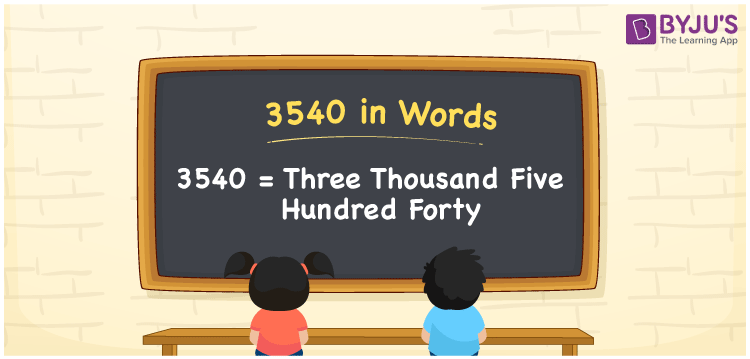# 3540 in words

3540 in words is written as Three Thousand Five Hundred and Forty. 3540 represents the count or value. The article on Counting Numbers can give you an idea about count or counting. The number 3540 is used in expressions that relate to money, distance, length, year and others. Let us consider an example for 3540. “You need to climb Three Thousand Five Hundred and Forty steps to visit a temple on the hill.” Another example, “There were 3540 un-read mails on a Monday Morning”.

 3540 in words Three Thousand Five Hundred and Forty Three Thousand Five Hundred and Forty in Numbers 3540

## 3540 in English Words## How to Write 3540 in Words?

We can convert 3540 to words using a place value chart. The number 3540 has 4 digits, so let’s make a chart that shows the place value up to 4 digits.

 Thousands Hundreds Tens Ones 3 5 4 0

Thus, we can write the expanded form as:

3 × Thousand + 5 × Hundred + 4 × Ten + 0 × One

= 3 × 1000 + 5 × 100 + 4 × 10 + 0 × 1

= 3540

= Three Thousand Five Hundred and Forty.

3540 is the natural number that is succeeded by 3539 and preceded by 3541.

3540 in words –Three Thousand Five Hundred and Forty.

Is 3540 an odd number? – No.

Is 3540 an even number? – Yes.

Is 3540 a perfect square number? – No.

Is 3540 a perfect cube number? – No.

Is 3540 a prime number? – No.

Is 3540 a composite number? – Yes.

## Solved Example

1. Write the number 3540 in expanded form

Solution: 3 × 1000 + 5 × 100 + 4 × 10 + 0 × 1.

We can write 3540 = 3000 + 500 + 40 + 0

= 3 × 1000 + 5 × 100 + 4 × 10 + 0 × 1.

## Frequently Asked Questions on 3540 in words

Q1

### How to write 3540 in words?

3540 in words is written as Three Thousand Five Hundred and Forty.
Q2

### State whether True or False. 3540 is divisible by 3?

True. 3540 is divisible by 3.
Q3

### Is 3540 divisible by 10?

Yes. 3540 is divisible 10.